The addition formulas for the sine and cosine are given by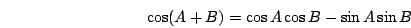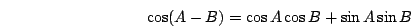The addition formulas for the tangent are given byEx 1 Findusing an addition formula.

Sol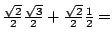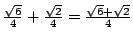.

Ex 2 Findusing an addition formula.

Sol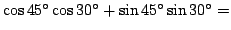.

Pr 1 Findusing an addition formula.

Pr 2 Findusing an addition formula.

Pr 3 Findusing an addition formula.

Pr 4 Use an addition formula to simplify.

Pr 5 Use an addition formula to simplify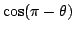.

Pr 6 Findusing an addition formula.

Pr 7 Use the addition formulas to simplify the expressionPr 8 Find the smallest positive angle between the lines with equationsand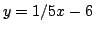.
Go to Solutions.

Lawrence Marx 2002-07-11